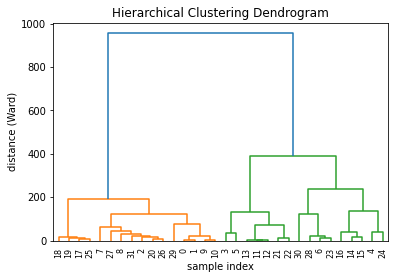To draw a dendrogram, you first need to have a numeric matrix. Each line represents an entity (here a car). Each column is a variable that describes the cars. The objective is to cluster the entities to show who shares similarities with whom. The dendrogram will draw the similar entities closer to each other in the tree.

``````# Libraries
import pandas as pd
from matplotlib import pyplot as plt
import numpy as np

# Import the mtcars dataset from the web + keep only numeric variables
url = 'https://raw.githubusercontent.com/holtzy/The-Python-Graph-Gallery/master/static/data/mtcars.csv'
df = df.set_index('model')
df = df.reset_index(drop=True)
mpg cyl disp hp drat wt qsec vs am gear carb
0 21.0 6 160.0 110 3.90 2.620 16.46 0 1 4 4
1 21.0 6 160.0 110 3.90 2.875 17.02 0 1 4 4
2 22.8 4 108.0 93 3.85 2.320 18.61 1 1 4 1
3 21.4 6 258.0 110 3.08 3.215 19.44 1 0 3 1
4 18.7 8 360.0 175 3.15 3.440 17.02 0 0 3 2

All right, now that we have our numeric matrix, we can calculate the distance between each car, and draw the hierarchical clustering. Distance calculation can be done by the `linkage()` function. I strongly advise you to visit the next page for more details concerning this crucial step.

``````# Calculate the distance between each sample
# You have to think about the metric you use (how to measure similarity) + about the method of clusterization you use (How to group cars)

Last but not least, you can easily plot this object as a dendrogram using the `dendrogram()` function of scipy library. These parameters are passed to the function:

• `Z` : The linkage matrix
• `labels` : Labels to put under the leaf node
• `leaf_rotation` : Specifies the angle (in degrees) to rotate the leaf labels

See post #401 for possible customisations to a dendrogram.

``````# Plot title
plt.title('Hierarchical Clustering Dendrogram')

# Plot axis labels
plt.xlabel('sample index')
plt.ylabel('distance (Ward)')

# Make the dendrogram
dendrogram(Z, labels=df.index, leaf_rotation=90)

# Show the graph
plt.show()``````## Contact & Edit

👋 This document is a work by Yan Holtz. You can contribute on github, send me a feedback on twitter or subscribe to the newsletter to know when new examples are published! 🔥